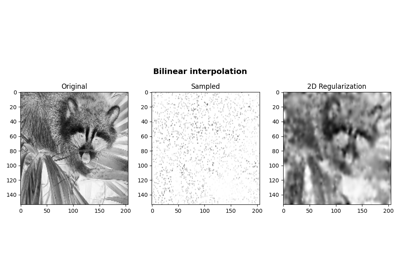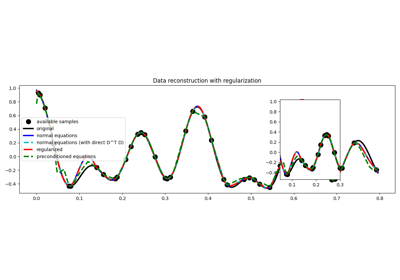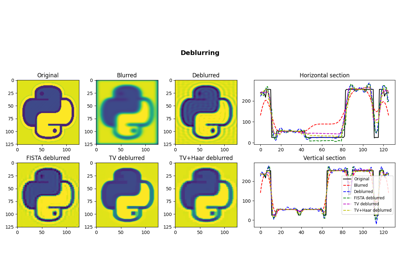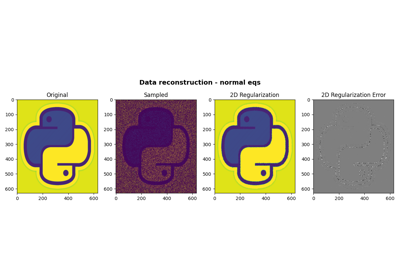# pylops.optimization.leastsquares.normal_equations_inversion#

pylops.optimization.leastsquares.normal_equations_inversion(Op, y, Regs, x0=None, Weight=None, dataregs=None, epsI=0.0, epsRs=None, NRegs=None, epsNRs=None, engine='scipy', show=False, **kwargs_solver)[source]#

Inversion of normal equations.

Solve the regularized normal equations for a system of equations given the operator Op, a data weighting operator Weight and optionally a list of regularization terms Regs and/or NRegs.

Parameters
Oppylops.LinearOperator

Operator to invert of size $$[N \times M]$$

ynumpy.ndarray

Data of size $$[N \times 1]$$

Regslist

Regularization operators (None to avoid adding regularization)

x0numpy.ndarray, optional

Initial guess of size $$[M \times 1]$$

Weightpylops.LinearOperator, optional

Weight operator

dataregslist, optional

Regularization data (must have the same number of elements as Regs)

epsIfloat, optional

Tikhonov damping

epsRslist, optional

Regularization dampings (must have the same number of elements as Regs)

NRegslist

Normal regularization operators (None to avoid adding regularization). Such operators must apply the chain of the forward and the adjoint in one go. This can be convenient in cases where a faster implementation is available compared to applying the forward followed by the adjoint.

epsNRslist, optional

Regularization dampings for normal operators (must have the same number of elements as NRegs)

enginestr, optional

Solver to use (scipy or pylops)

showbool, optional

Display normal equations solver log

**kwargs_solver

Arbitrary keyword arguments for chosen solver (scipy.sparse.linalg.cg and pylops.optimization.solver.cg are used for engine scipy and pylops, respectively)

Note

When user does not supply atol, it is set to “legacy”.

Returns
xinvnumpy.ndarray

Inverted model.

istopint

Convergence information (only when using scipy.sparse.linalg.cg):

0: successful exit

>0: convergence to tolerance not achieved, number of iterations

<0: illegal input or breakdown

RegularizedInversion

Regularized inversion

PreconditionedInversion

Preconditioned inversion

Notes

## Examples using pylops.optimization.leastsquares.normal_equations_inversion#Bilinear Interpolation

Bilinear Interpolation03. Solvers

03. Solvers05. Image deblurring

05. Image deblurring06. 2D Interpolation

06. 2D Interpolation